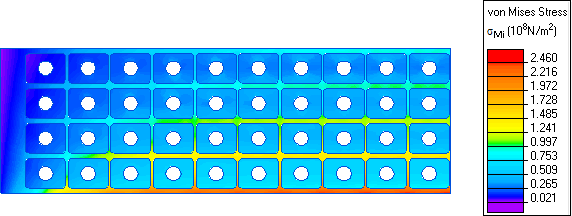QuickField

A new approach to field modelling

Main >> >> Tokamak solenoid simulation

# TOKAMAK solenoid mechanical stress

QuickField simulation example

The central solenoid of the ohmic heating system for a TOKAMAK fusion device.

Problem Type
Axisymmetric multiphysics problem of DC magnetics coupled to Stress analysis.

Geometry

Given
Current density in coils j = 3·108 A/m²;
Magnetic permittivity of plastic, coils and liquid helium inside coils μ = 1.

Copper of coils:
Young's modulus E = 7.74·1010 N/m²;
Poisson's ratio ν = 0.335;
Maximum allowable stress: 2.2·108 N/m².

Plastic structure:
Young's modulus E = 2·1011 N/m²;
Poisson's ratio ν = 0.35;
Maximum allowable stress: 109 N/m².

Solution

The solenoid consists of 80 superconducting coils fixed in common plastic structure. Due to mirror symmetry one half of the structure is modeled.

The Coupl4MS.pbm is the problem of calculating the magnetic field generated by the solenoid, and Coupl4SA.pbm analyzes stresses and deformations in coils and plastic structure due to Lorentz forces acting on the coils.

Results
Magnetic flux density distribution in TOKAMAK solenoid:Mechanical stress distribution in TOKAMAK solenoid: